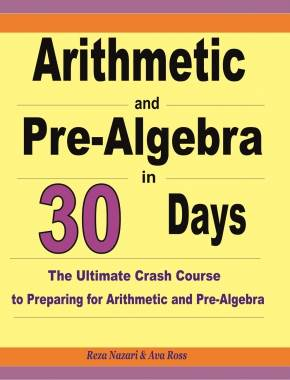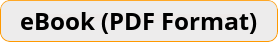\$11.99

# Arithmetic and Pre-Algebra in 30 Days The Ultimate Crash Course to Preparing for Arithmetic and Pre-AlgebraArithmetic and Pre-Algebra in 30 Days helps you learn all Math topics that you will need to prepare for the Arithmetic and Pre-Algebra exam. You only need to spend about 90–120 minutes daily in your 30–day period in order to ace the Arithmetic and Pre-Algebra exam. This book with more than 2,500 math questions is all you will ever need to fully prepare for the Arithmetic and Pre-Algebra.Teacher's Choice
100% Guaranteed
Secure Checkout

The Best Book to Prepare for Arithmetic and Pre-Algebra Exam!

The goal of this book is simple. It will help you incorporate the best method and the right strategies to prepare for the Arithmetic and Pre-Algebra exam FAST and EFFECTIVELY.

Arithmetic and Pre-Algebra in 30 Days helps you learn all Math topics that you will need to prepare for the Arithmetic and Pre-Algebra exam. You only need to spend about 90–120 minutes daily in your 30–day period in order to ace the Arithmetic and Pre-Algebra exam. This book with more than 2,500 math questions is all you will ever need to fully prepare for the Arithmetic and Pre-Algebra.

Arithmetic and Pre-Algebra in 30 Days provides students with the confidence and math skills they need to succeed on the Arithmetic and Pre-Algebra exams, providing a solid foundation of basic Math topics with abundant exercises for each topic. It is designed to address the needs of Arithmetic and Pre-Algebra takers who must have a working knowledge of basic Math.

Whether you are intimidated by math, or even if you were the first to raise your hand in the Math classes, this book can help you accelerate the learning process and put you on the right track.

Inside the pages of this comprehensive book, students can learn basic math operations in a structured manner with a complete study program to help them understand essential math skills. It also has many exciting features, including:

• Dynamic design and easy–to–follow activities
• Step–by–step guide for all Math topics
• Targeted, skill–building practices
• A fun, interactive and concrete learning process
• Math topics are grouped by category, so you can focus on the topics you struggle on
• All solutions for the exercises are included, so you will always find the answers

Arithmetic and Pre-Algebra in 30 Days is for all Arithmetic and Pre-Algebra course takers. It is a breakthrough in Math learning — offering a winning formula and the most powerful methods for learning basic Math topics confidently. Each section offers step–by–step instruction and helpful hints, with a few topics being tackled each day.

Effortlessly and confidently follow the step–by–step instructions in this book to prepare for the Arithmetic and Pre-Algebra in a short period of time.

Arithmetic and Pre-Algebra in 30 Days is the only book you’ll ever need to master Basic Math topics! It can be used as a self–study course – you do not need to work with a Math tutor. (It can also be used with a Math tutor).

You’ll be surprised how fast you master the Math topics covering on Arithmetic and Pre-Algebra.

Ideal for self–study as well as for classroom usage.